Vol. Practical Guide to Radio-Frequency Analysis and Design

# How to Demodulate an FM Waveform

## Learn about two techniques for recovering the baseband signal from a frequency-modulated carrier.

Learn about two techniques for recovering the baseband signal from a frequency-modulated carrier.

Frequency modulation offers improved performance over amplitude modulation, but it is somewhat more difficult to extract the original information from an FM waveform. There are a few different ways to demodulate FM; in this page we’ll discuss two. One of these is quite straightforward, and the other is more complex.

### Creating the Signal

As in How to Demodulate an AM Waveform, we’ll use LTspice to explore FM demodulation, and once again we need to first perform frequency modulation so that we have something to demodulate. If you look back at the page on analog frequency modulation, you will see that the mathematical relationship is less straightforward than that of amplitude modulation. With AM, we simply added an offset and then performed ordinary multiplication. With FM, we need to add continuously varying values to the quantity inside a sine (or cosine) function, and furthermore, these continuously varying values are not the baseband signal but rather the integral of the baseband signal.

Consequently, we can’t generate an FM waveform using an arbitrary behavioral voltage source and a simple mathematical relationship, as we did with AM. It turns out, though, that it is actually easier to generate an FM signal. We simply use the SFFM option for a normal voltage source: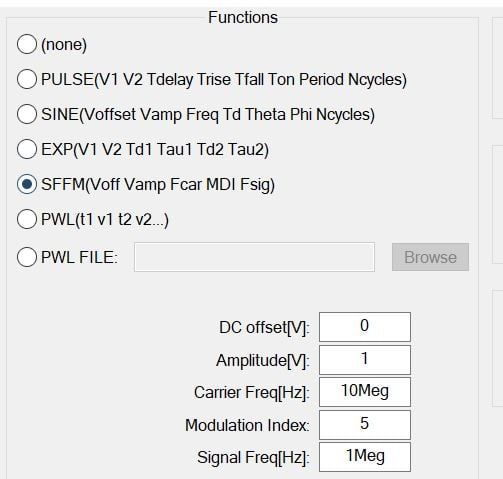The following “circuit” is all we need for creating an FM waveform consisting of a 10 MHz carrier and a 1 MHz sinusoidal baseband signal: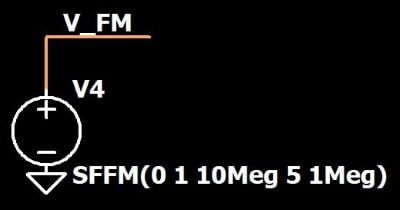Note that the modulation index is five; a higher modulation index makes it easier to see the frequency variations. The following plot shows the waveform created by the SFFM voltage source.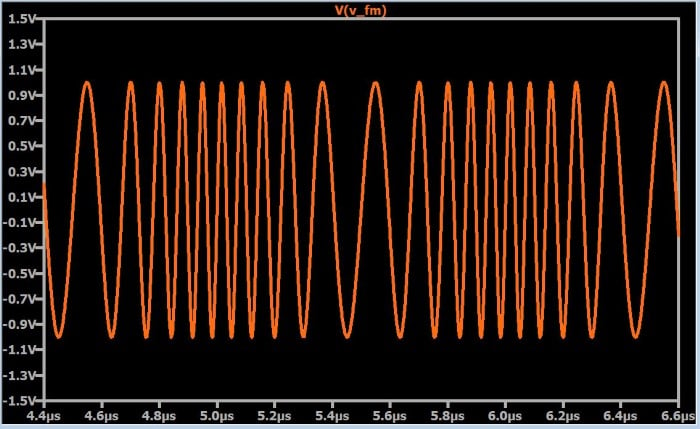### Demodulation: The High-Pass Filter

The first demodulation technique that we’ll look at begins with a high-pass filter. We’ll assume that we’re dealing with narrowband FM (which is discussed briefly in this page). We need to design the high-pass filter such that the attenuation will vary significantly within a frequency band whose width is twice the bandwidth of the baseband signal. Let’s explore this concept more thoroughly.

The received FM signal will have a spectrum that is centered around the carrier frequency. The width of the spectrum is approximately equal to twice the bandwidth of the baseband signal; the factor of two results from the shifting of the positive and negative baseband frequencies (as discussed here), and it is “approximately” equal because the integration applied to the baseband signal can affect the shape of the modulated spectrum. Thus, the lowest frequency in the modulated signal is approximately equal to the carrier frequency minus the highest frequency in the baseband signal, and the highest frequency in the modulated signal is approximately equal to the carrier frequency plus the highest frequency in the baseband signal.

Our high-pass filter needs to have a frequency response that causes the lowest frequency in the modulated signal to be attenuated significantly more than the highest frequency in the modulated signal. If we apply this filter to an FM waveform, what will be the result? It will be something like this: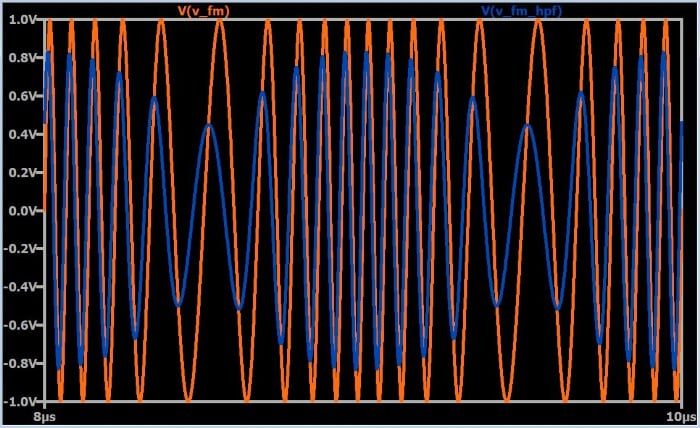This plot shows both the original FM waveform and the high-pass-filtered waveform, for purposes of comparison. The next plot shows just the filtered waveform, so that you can see it more clearly.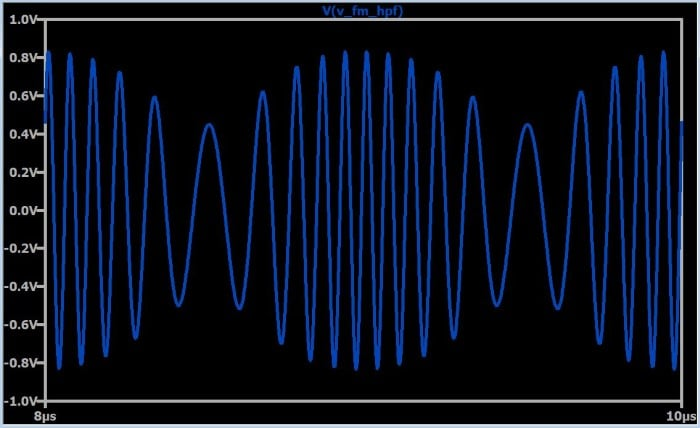By applying the filter, we have turned frequency modulation into amplitude modulation. This is a convenient approach to FM demodulation, because it allows us to benefit from envelope-detector circuitry that has been developed for use with amplitude modulation. The filter used to produce this waveform was nothing more than an RC high-pass with a cutoff frequency approximately equal to the carrier frequency.

#### Amplitude Noise

The simplicity of this demodulation scheme naturally makes us think that it is not the highest-performance option, and in fact this approach does have a major weakness: it is sensitive to amplitude variations. The transmitted signal will have a constant envelope because frequency modulation does not involve changes to the amplitude of the carrier, but the received signal will not have a constant envelope because amplitude is inevitably affected by error sources.

Consequently, we cannot design an acceptable FM demodulator simply by adding a high-pass filter to an AM demodulator. We also need a limiter, which is a circuit that mitigates amplitude variations by restricting the received signal to a certain amplitude. The existence of this simple and effective remedy for amplitude variations enables FM to maintain its greater (compared to AM) robustness against amplitude noise: We cannot use a limiter with AM signals because restricting the amplitude corrupts the information encoded in the carrier. FM, on the other hand, encodes all of the information in the temporal characteristics of the transmitted signal.

### Demodulation: The Phase-Locked Loop

A phase-locked loop (PLL) can be used to create a complex but high-performance circuit for FM demodulation. A PLL can “lock onto” the frequency of an incoming waveform. It does this by combining a phase detector, a low-pass filter (aka “loop filter”), and a voltage-controlled oscillator (VCO) into a negative-feedback system, as follows: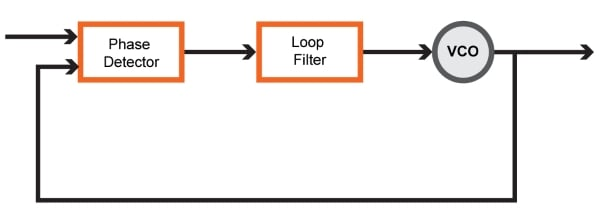After the PLL has locked, it can create an output sinusoid that follows frequency variations in the incoming sinusoid. This output waveform would be taken from the output of the VCO. In an FM-demodulator application, however, we don’t need an output sinusoid that has the same frequency as the input signal. Instead, we use the output from the loop filter as a demodulated signal. Let’s look at why this is possible.

The phase detector produces a signal that is proportional to the phase difference between the incoming waveform and the output of the VCO. The loop filter smooths this signal, which then becomes the control signal for the VCO. Thus, if the frequency of the incoming signal is constantly increasing and decreasing, the VCO control signal has to increase and decrease accordingly to ensure that the VCO output frequency remains equal to the input frequency. In other words, the output of the loop filter is a signal whose amplitude variations correspond to the input-frequency variations. This is how a PLL accomplishes frequency demodulation.

### Summary

• In LTspice, a frequency-modulated sinusoid can be generated by using the SFFM option for standard voltage sources.
• A simple and effective FM demodulation technique involves a high-pass filter (for FM-to-AM conversion) followed by an AM demodulator.
• A high-pass-filter-based FM demodulator is preceded by a limiter to prevent amplitude variations from contributing error to the demodulated signal.
• A phase-locked loop can be used to achieve high-performance FM demodulation. The use of integrated-circuit PLLs makes this approach less complex than it might seem.

#### Lessons in Electric Circuits

##### Volumes »
Published under the terms and conditions of the Design Science License
1 Comment•lgunther January 08, 2022

Please specify completely the shape of the high pass filter. Including only n=1, 2, 3, ...  is not good at all.  n=2,... is not good: While it yields an amplitude modulation, the modulation is not sinusoidal.
Using a low pass filter, with n=-1, -2, ... isn’t OK either.

Like.